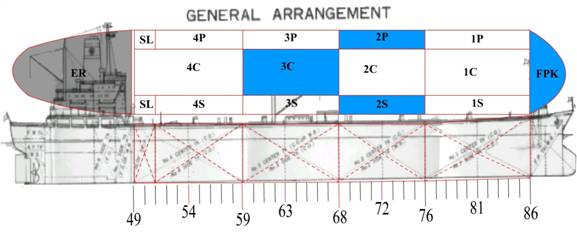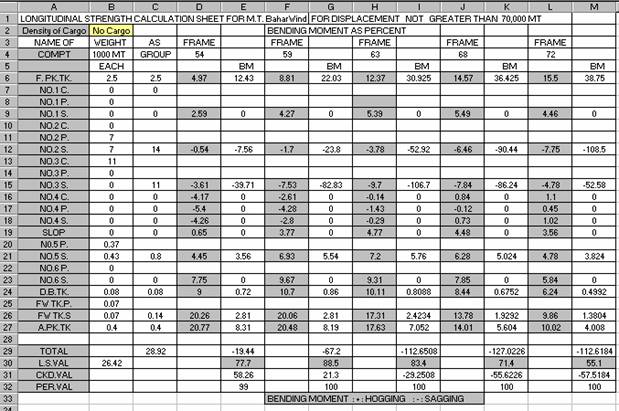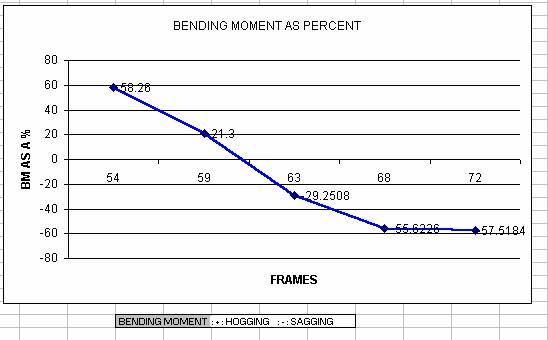Back to Nautical Ship Form Stresses Hull Structure Bow & Stern Load Lines Rudder & Propeller

Ship Construction

Ship Stresses

Shear force and bending moments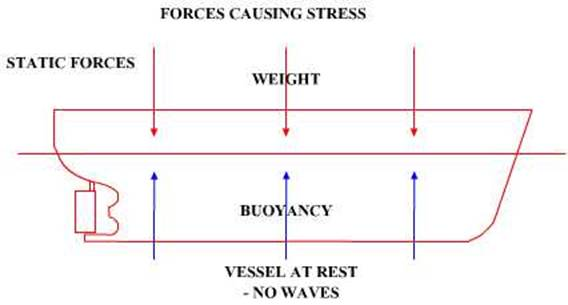When a section such as a beam is carrying a load there is a tendency for some parts to be pushed upwards and for other parts to move downwards, this tendency is termed Shearing.

The Shear force at a point or station is the vertical force at that point. The shear force at a station may also defined as being the total load on either the left hand side or the right hand side of the station; load being defined as the difference between the down and the upward forces, or for a ship the weight would be the downward force and the buoyancy would be the upward thrust or force.

The longitudinal stresses imposed by the weight and buoyancy distribution may give rise to longitudinal shearing stresses. The maximum shearing stress occurs at the neutral axis and a minimum at the deck and keel. Vertical shearing stresses may also occur.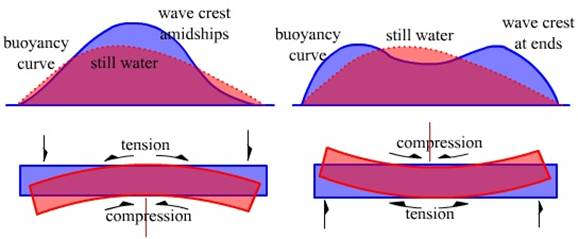Bending Moment

The beam, which we have been considering, would also have a tendency to bend and the bending moment measures this tendency.

Its size depends upon the amount of the load as well as how the load is placed together with the method of support.

Bending moments are calculated in the same way as ordinary moments that is multiplying force by distance, and so they are expressed in weight – length units.

As with the calculation of shear force the bending moment at a station is obtained by considering moments either to the left or to the right of the station.

Hogging and sagging

Hogging – When a beam is loaded or other wise is subjected to external forces such that the beam bends with the ends curving downwards it is termed as hogging stress.

For a ship improper loading as well as in a seaway when riding the crest of a wave the unsupported ends of the ship would have a tendency similar to the beam above.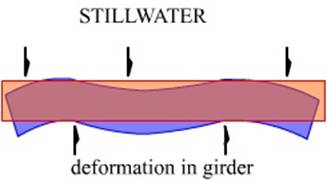Sagging – In this case the beam is loaded or other wise subjected to external forces making the beam bend in such a way that the ends curve upwards, this is termed as sagging.

Similar with a ship if improper loaded or when riding the trough of a wave – with crests at both ends then the ship is termed to be sagging.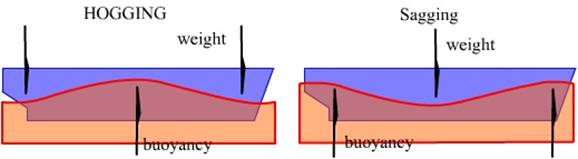For Hogging the ship ends to curve downwards would mean that the weight/ load amidships is much less than at the end holds/ tanks.

For Sagging the ship would have been loaded in such a manner that a greater percentage of the load is around the midship area.

In a seaway the hogging and the sagging stresses are amplified when riding the crests and falling into the troughs. Thus especially for large ships there are two conditions in the stability software – Sea Condition and Harbour condition.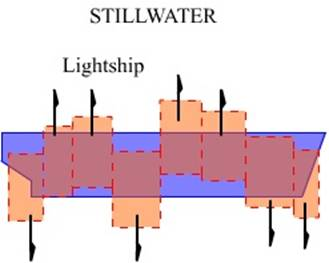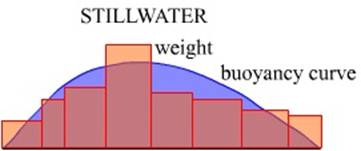A ship loaded while set in the harbour condition may allow loading with hogging/ sagging stresses reaching a high level, when this state of loading is transferred to a Sea condition in the software the results would be catastrophic since now the wave motions have also been incorporated.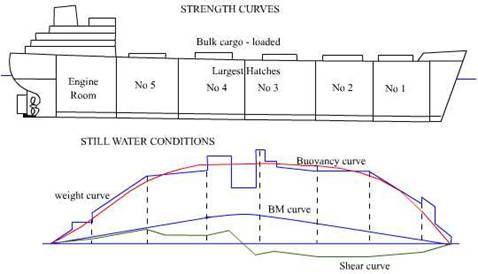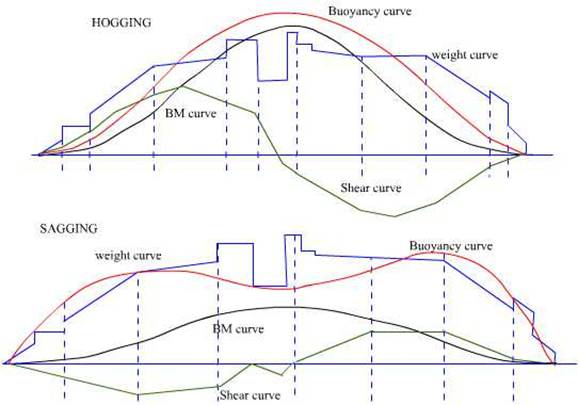Discharging in port may be planned in the Harbour Condition.

Hogging and sagging cause compressive and tensile stresses on the ship beam – notably on the deck and the keel structure.

Water pressure and Thrust

Pressure is force per unit area and water pressure is dependent on the head of the water column affecting the point of the measurement of the pressure.

Let us assume an area of 1sq.m. then this area of water up to a depth of 1 m below the surface would have a volume of 1sq.m. x 1m = 1cbm and the weight of this volume would be 1cbm x density of the water = 1MT (assuming that it is FW) or 1000kgf, therefore the pressure exerted by this mass would be 1000kgf/sq.m.

Similarly if now the depth of measurement is increased to 3m then the volume of this area subtending up to the 3m mark would be 1sq.m x 3 = 3cbm and the weight of the water would be 3MT or 3000kgf and the pressure exerted would be 3000kgf/sq.m.

If now the liquid had not been FW but any other then the weight would be found by multiplying the volume by the density of the liquid. And thus the pressure exerted would be found.

If we now increase the area of the square of water plane would it make a difference in the pressure?

Let us consider a area of 2000sq.m then the volume of this water at a depth of 1 m would be 2000cbm and the weight would be 2000MT (consider FW) and the pressure exerted would be 2000,000kgf/ 2000sq.m which would give us again 1000kgf/sqm, thus the pressure is independent of the area of the water plane.

Thrust however is different, thrust is taken to be the total weight of the liquid over an area. Thus for the previous example the thrust would be 2000 tonnes.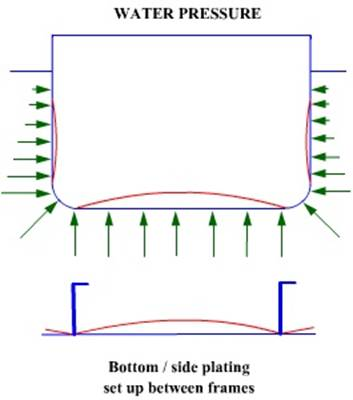Thus the thrust is given by: the area of the water plane x pressure head x density of the liquid.

Thrust always acts at right angles to the immersed surface and for any depth the thrust in any of the directions is the same. The pressure head which is used in the above calculation of thrust is the depth of the geometrical centre of the area below the surface of the liquid.

For a ship the thrust on the ship side changes as the depth increases, however the bottom is affected uniformly for a set depth.

Centre of pressure of an area is the point on the area where the thrust could be considered to act. It is taken that the centre of pressure is at 2/3rds the depth below the surface for ordinary vertical bulkheads and at half the depth in the case of collision bulkheads.

Racking stress and its causes

In a seaway as a ship rolls from one side to the other the different areas of the ship have motion which are dependent on the nature of the subject area. The accelerations are thus not similar due to the various masses of the different sections (although joined together). These accelerations on the ships structure are liable to cause distortion in the transverse section. The greatest effect is under light ship conditions.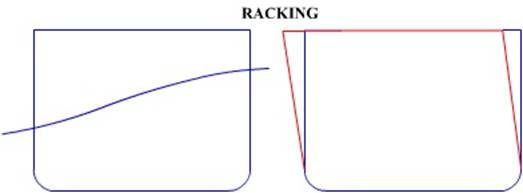Local Stresses

Panting

This is a stress, which occurs at the ends of a vessel due to variations in water pressure on the shell plating as the vessel pitches in a seaway. The effect is accentuated at the bow when making headway.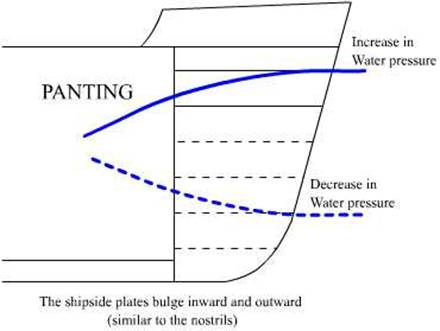Pounding:

Heavy pitching assisted by heaving as the whole vessel is lifted in a seaway and again as the vessel slams down on the water is known as pounding or slamming. This may subject the forepart to severe blows from the sea. The greatest effect is experienced in the light ship condition.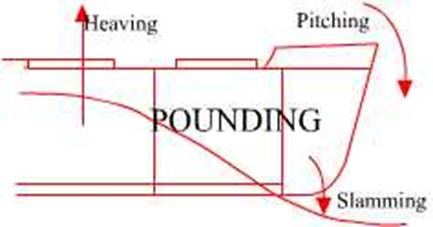Localized heavy loads may give rise to localized distortion of the transverse section.

Such local loads may be the machinery (Main engine) in the engine room or the loading of concentrated ore in the holds.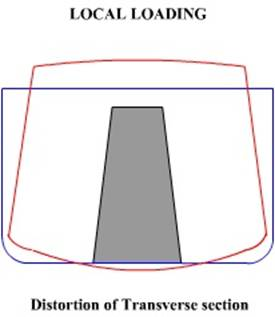Shearing force Curve

The following example shown is for an old tanker in the ballast condition.

The compartments loaded are the Fpk tank, WB tanks 2P and 2S, WB tank 3C and other miscellaneous tanks in the after section of the tanker.

The SF is calculated as per the manual with the multipliers having been set by the shipyard and approved by the classification society.

If we are to assume that the ship is a beam then the loads are at the fore end – midship region and the after section which has the accommodation as well as the ER.

The SF curve is reproduced and the maximum occur at frames 54 and between 68 to 72, this corresponds to the area on the ship – mid 4C and between 2C (aft to mid region). Note that the signs have changed between the frames 54 and 68 with a point between frames 59 to 63 (3C mid to aft) registering 0 value.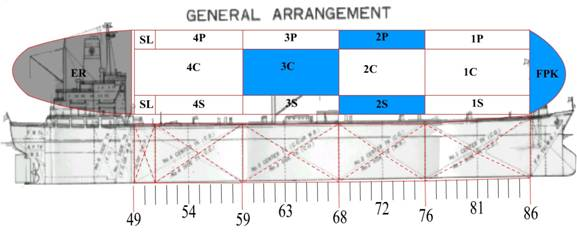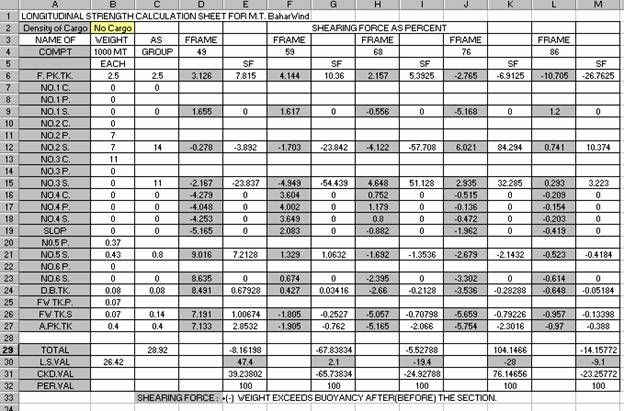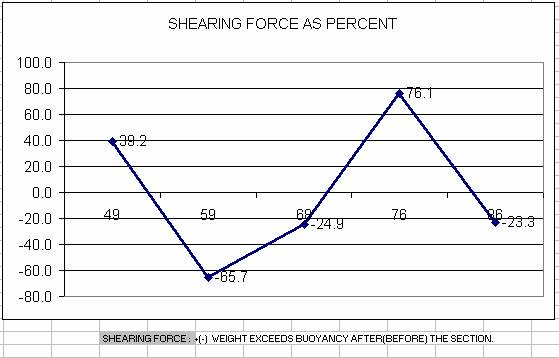Bending Moment Curve

The following example shown is for a old tanker in the ballast condition.

The compartments loaded are the Fpk tank, WB tanks 2P and 2S, WB tank 3C and other miscellaneous tanks in the after section of the tanker.

The BM is calculated as per the manual with the multipliers having been set by the shipyard and approved by the classification society.

If we are to assume that the ship is a beam then the loads are at the fore end – midship region and the after section which has the accommodation as well as the ER.

The BM curve is reproduced and the maximum occur at frames 59 and 76, this corresponds to the area on the ship – 4C forward bulkhead and 2C forward bulkhead. Note that the signs have changed twice.